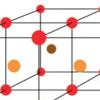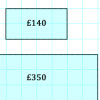Search by Topic

Resources tagged with Creating and manipulating expressions and formulae similar to Smaller and Smaller:

Filter by: Content type:
Age range:
Challenge level:

There are 55 results

Broad Topics > Algebraic expressions, equations and formulae > Creating and manipulating expressions and formulaeCan They Be Equal?

Age 11 to 14 Challenge Level:

Can you find rectangles where the value of the area is the same as the value of the perimeter?Special Sums and Products

Age 11 to 14 Challenge Level:

Find some examples of pairs of numbers such that their sum is a factor of their product. eg. 4 + 12 = 16 and 4 × 12 = 48 and 16 is a factor of 48.Sum Equals Product

Age 11 to 14 Challenge Level:

The sum of the numbers 4 and 1 [1/3] is the same as the product of 4 and 1 [1/3]; that is to say 4 + 1 [1/3] = 4 � 1 [1/3]. What other numbers have the sum equal to the product and can this be so for. . . .Age 11 to 14 Challenge Level:

Visitors to Earth from the distant planet of Zub-Zorna were amazed when they found out that when the digits in this multiplication were reversed, the answer was the same! Find a way to explain. . . .The Number Jumbler

Age 7 to 14 Challenge Level:

The Number Jumbler can always work out your chosen symbol. Can you work out how?Age 11 to 14 Challenge Level:

Think of a number, add one, double it, take away 3, add the number you first thought of, add 7, divide by 3 and take away the number you first thought of. You should now be left with 2. How do I. . . .Magic Sums and Products

Age 11 to 16

How to build your own magic squares.Chocolate Maths

Age 11 to 14 Challenge Level:

Pick the number of times a week that you eat chocolate. This number must be more than one but less than ten. Multiply this number by 2. Add 5 (for Sunday). Multiply by 50... Can you explain why it. . . .Always the Same

Age 11 to 14 Challenge Level:

Arrange the numbers 1 to 16 into a 4 by 4 array. Choose a number. Cross out the numbers on the same row and column. Repeat this process. Add up you four numbers. Why do they always add up to 34?Magic Squares for Special Occasions

Age 11 to 16

This article explains how to make your own magic square to mark a special occasion with the special date of your choice on the top line.Perimeter Expressions

Age 11 to 14 Challenge Level:

Create some shapes by combining two or more rectangles. What can you say about the areas and perimeters of the shapes you can make?Multiply the Addition Square

Age 11 to 14 Challenge Level:

If you take a three by three square on a 1-10 addition square and multiply the diagonally opposite numbers together, what is the difference between these products. Why?Regular Hexagon Loops

Age 11 to 14 Challenge Level:

Make some loops out of regular hexagons. What rules can you discover?More Mathematical Mysteries

Age 11 to 14 Challenge Level:

Write down a three-digit number Change the order of the digits to get a different number Find the difference between the two three digit numbers Follow the rest of the instructions then try. . . .Is it Magic or Is it Maths?

Age 11 to 14 Challenge Level:

Here are three 'tricks' to amaze your friends. But the really clever trick is explaining to them why these 'tricks' are maths not magic. Like all good magicians, you should practice by trying. . . .Algebra from Geometry

Age 11 to 16 Challenge Level:

Account of an investigation which starts from the area of an annulus and leads to the formula for the difference of two squares.Age 11 to 14 Challenge Level:

A little bit of algebra explains this 'magic'. Ask a friend to pick 3 consecutive numbers and to tell you a multiple of 3. Then ask them to add the four numbers and multiply by 67, and to tell you. . . .Legs Eleven

Age 11 to 14 Challenge Level:

Take any four digit number. Move the first digit to the end and move the rest along. Now add your two numbers. Did you get a multiple of 11?Even So

Age 11 to 14 Challenge Level:

Find some triples of whole numbers a, b and c such that a^2 + b^2 + c^2 is a multiple of 4. Is it necessarily the case that a, b and c must all be even? If so, can you explain why?Hot Pursuit

Age 11 to 14 Challenge Level:

I added together the first 'n' positive integers and found that my answer was a 3 digit number in which all the digits were the same...Age 11 to 14 Challenge Level:

List any 3 numbers. It is always possible to find a subset of adjacent numbers that add up to a multiple of 3. Can you explain why and prove it?Age 11 to 14 Challenge Level:

Think of a number and follow my instructions. Tell me your answer, and I'll tell you what you started with! Can you explain how I know?Cubes Within Cubes Revisited

Age 11 to 14 Challenge Level:

Imagine starting with one yellow cube and covering it all over with a single layer of red cubes, and then covering that cube with a layer of blue cubes. How many red and blue cubes would you need?Boxed In

Age 11 to 14 Challenge Level:

A box has faces with areas 3, 12 and 25 square centimetres. What is the volume of the box?Algebra Match

Age 11 to 16 Challenge Level:

A task which depends on members of the group noticing the needs of others and responding.Crossed Ends

Age 11 to 14 Challenge Level:

Crosses can be drawn on number grids of various sizes. What do you notice when you add opposite ends?How Much Can We Spend?

Age 11 to 14 Challenge Level:

A country has decided to have just two different coins, 3z and 5z coins. Which totals can be made? Is there a largest total that cannot be made? How do you know?Finding 3D Stacks

Age 7 to 11 Challenge Level:

Can you find a way of counting the spheres in these arrangements?More Number Pyramids

Age 11 to 14 Challenge Level:

When number pyramids have a sequence on the bottom layer, some interesting patterns emerge...Christmas Chocolates

Age 11 to 14 Challenge Level:

How could Penny, Tom and Matthew work out how many chocolates there are in different sized boxes?Price Match

Age 7 to 11 Challenge Level:

Can you find pairs of differently sized windows that cost the same?Seven Squares

Age 11 to 14 Challenge Level:

Watch these videos to see how Phoebe, Alice and Luke chose to draw 7 squares. How would they draw 100?Always a Multiple?

Age 11 to 14 Challenge Level:

Think of a two digit number, reverse the digits, and add the numbers together. Something special happens...Hallway Borders

Age 11 to 14 Challenge Level:

What are the possible dimensions of a rectangular hallway if the number of tiles around the perimeter is exactly half the total number of tiles?Age 7 to 14 Challenge Level:

Think of a number and follow the machine's instructions... I know what your number is! Can you explain how I know?Good Work If You Can Get It

Age 11 to 14 Challenge Level:

A job needs three men but in fact six people do it. When it is finished they are all paid the same. How much was paid in total, and much does each man get if the money is shared as Fred suggests?Number Pyramids

Age 11 to 14 Challenge Level:

Try entering different sets of numbers in the number pyramids. How does the total at the top change?Think of Two Numbers

Age 11 to 14 Challenge Level:

Think of two whole numbers under 10, and follow the steps. I can work out both your numbers very quickly. How?Partitioning Revisited

Age 11 to 14 Challenge Level:

We can show that (x + 1)² = x² + 2x + 1 by considering the area of an (x + 1) by (x + 1) square. Show in a similar way that (x + 2)² = x² + 4x + 4Why 8?

Age 11 to 14 Challenge Level:

Choose any four consecutive even numbers. Multiply the two middle numbers together. Multiply the first and last numbers. Now subtract your second answer from the first. Try it with your own. . . .Card Trick 1

Age 11 to 14 Challenge Level:

Can you explain how this card trick works?Age 11 to 14 Challenge Level:

Think of a number Multiply it by 3 Add 6 Take away your start number Divide by 2 Take away your number. (You have finished with 3!) HOW DOES THIS WORK?Temperature

Age 11 to 14 Challenge Level:

Is there a temperature at which Celsius and Fahrenheit readings are the same?Summing Consecutive Numbers

Age 11 to 14 Challenge Level:

15 = 7 + 8 and 10 = 1 + 2 + 3 + 4. Can you say which numbers can be expressed as the sum of two or more consecutive integers?Fibs

Age 11 to 14 Challenge Level:

The well known Fibonacci sequence is 1 ,1, 2, 3, 5, 8, 13, 21.... How many Fibonacci sequences can you find containing the number 196 as one of the terms?Sums of Pairs

Age 11 to 16 Challenge Level:

Jo has three numbers which she adds together in pairs. When she does this she has three different totals: 11, 17 and 22 What are the three numbers Jo had to start with?”Special Numbers

Age 11 to 14 Challenge Level:

My two digit number is special because adding the sum of its digits to the product of its digits gives me my original number. What could my number be?Quick Times

Age 11 to 14 Challenge Level:

32 x 38 = 30 x 40 + 2 x 8; 34 x 36 = 30 x 40 + 4 x 6; 56 x 54 = 50 x 60 + 6 x 4; 73 x 77 = 70 x 80 + 3 x 7 Verify and generalise if possible.Top-heavy Pyramids

Age 11 to 14 Challenge Level:

Use the numbers in the box below to make the base of a top-heavy pyramid whose top number is 200.How Many Miles to Go?

Age 11 to 14 Challenge Level:

How many more miles must the car travel before the numbers on the milometer and the trip meter contain the same digits in the same order?